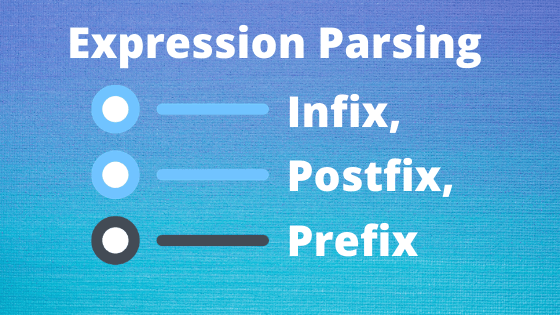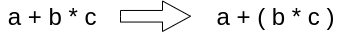# Expression Parsing Infix, Postfix, and Prefix## Expression Parsing (Infix, Prefix and Postfix)

Any arithmetic expression can be written in three different but equal notations, i.e. without modifying an expression’s meaning or output.
Those notations are −
• Infix Notation
• Prefix (Polish) Notation
• Postfix (Reverse-Polish) Notation

## Expression Parsing

There are 3 types of notation we read in the first paragraph. We will read each notation in details as well as how to convert

### 4. Tree From Postfix

but before that we will know what is Precedence and Associativity.

### Precedence

Precedence means giving the priority and more importance than else. Suppose an operand is in between two separate operators, which operator must take the operand first, is determined by the operator’s precedence over others. For example –Since the operation of multiplication takes precedence over the addition, b* c is evaluated first. Later, an operator precedence table will be given.

### Associativity

Associativity defines the concept where operators occur in an expression with the same precedence. For example, in expression a + b − c, both + and – have the same precedence, then the associativity of such operators determines which part of the expression will be evaluated first. Both + and − are left associative here, so the expression will be calculated as (a+b) −c.

Precedence and associativity define the order in which an expression is evaluated.
Sr.NoOperatorPrecedenceAssociativity
1Exponentiation ^HighestRight Associative
2Multiplication ( * ) and Division ( / ) Second HighestLeft Associative
3Addition ( + ) and Subtraction ( – ) LowestLeft Associative
The above table describes the default behavior of operators. The order can be modified at any stage in the expression evaluation, using parenthesis. For example  −
In x + y * z,  the expression element y*z is evaluated first, with the precedence of multiplication over addition. We use parenthesis here for first evaluation of x + y, like (x+y)*z

## What is an infix, prefix, and postfix expression?

Prefix : –  (Pre = के पहले) Meaning when the operation in an expression comes before the operand it is called prefix.
General Syntex =
example : +AC

Infix :- (In = बीच में ) When the operation in an expression is between two operands, it is called infix.
General Syntex =
example : A + C

Postfix :- (Post = बाद में  ) When operation comes after operand in an expression, it is called postfix.
General Syntex =
example : AC+

## Infix To Postfix Conversion Using STACK

### What are the rules of infix to postfix conversion?

Following the rules, we have to follow :

1. Print operands as they arrive
2. if the stack is empty or has left parenthesis on top, push the incoming operator onto the stack
3. An incoming symbol is ‘(‘, then push it onto the stack
4. If the received symbol is ‘) ‘ the stack will pop up and print the operators before left parenthesis is found
5. The upcoming symbol has higher precedence then the top of the stack, push it on the stack.
6. If the next symbol has lower precedence than the top of the stack, pop, and print the top. Then test the incoming operator against the new top of the stack.
7. If the arriving operator has equal precedence with top of the stack, use associativity rule(a,b)
8. pop and print all

Note: (associativity rule)
(a) Associativity L to R then pop and print the top of the stack and then push the incoming operator.
(b) if associativity R to L then push the incoming operator.

###### Let’s take an example of Infix to Postfix for better understand

Infix Expression: A + B * C / D – E

S.NoScannedSTACKPostfix ExpressionDescription
1AEMPTYASTART
2++A
3B+AB
4*+ *ABRULE NO 5, BECAUSE ‘*’ HAS HIGHER PRECEDENCE
5C+ *ABC
6/+ /ABC*FIRST APPLY RULE NO 7, AND THAN 5
7D+/ABC*D
8ABC*D/+APPLY RULE NO. 6
9EABC*D/+E
10ABC*D/+E-APPLY RUL NO 8

Resultant Postfix Expression: ABC*D/+E –

## Infix To Prefix Using Stack

For infix to prefix, we use same rules but must reverse the expression. For example A + B / C, here we reverse this expression like  C / B + A then applies all those rules which are applicable on infix to postfix.